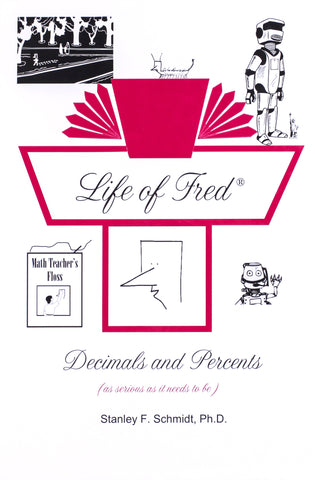Life of Fred: Decimals and Percents

• ₱1,200.00

This book covers the second half of the introduction to pre-algebra (after Life of Fred: Fractions). After this book you are ready for pre-algebra.

Read about one afternoon and evening in Fred's life in which he:
Builds a robot
Gets his first pet
Learns why sea chanties are not popular in some boat rental stores

This book covers second half of the introduction to pre-algebra including . . .

• Number Systems
• Multiplying by Ten
• Functions and Inverse Functions
• Pi
• Multiplying Decimals
• Whole Numbers
• Squaring a Number
• Subtracting Mixed Units
• Sets and Subsets
• Union and Intersection of Sets
• Rules of Divisibility
• Division of Decimals
• Repeating Decimals
• Bar Graphs
• Prime and Composite Numbers
• Consecutive Numbers
• The Goldbach Conjecture
• Area of a Circle
• Dollars vs. Cents
• Pie Charts
• Conversion between Percents/ Fractions/Decimals
• 40% of 15
• 30% off
• Distance Equals Rate Times Time
• 15% more
• Area of a Triangle
• Square Roots
• Area of a Parallelogram
• 13 Is What Percent of 52
• Ratio
• Ordered Pairs
• Graphing
• Negative Numbers
• Elapsed Time
• Probability
• And more . . .

Unlike all other math programs, this one also teaches about:
When Lincoln used the vigesimal system
When robots have tea parties
How flossing helped Fred to read books in bed at night

The answers are included in the textbook.

Life of Fred Decimals and Percents is a hardcover textbook containing 192 pages. This book is not consumable. All answers are written on separate paper or in a notebook.

(description c/o the Life of Fred website)

We Also Recommend